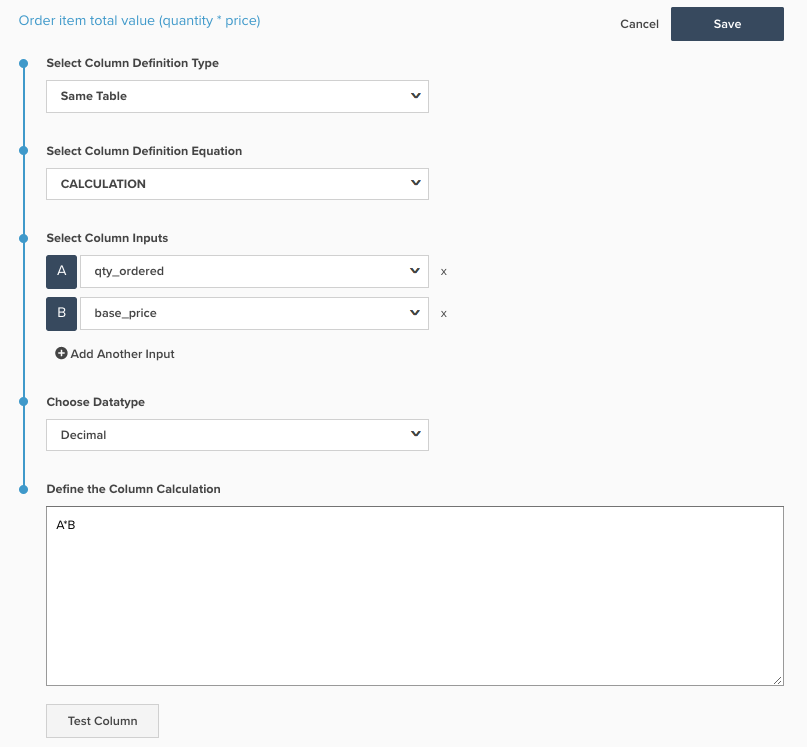# Create a SQL Calculated Column

This topic outlines the purpose and uses of the `Calculation` column type, that can be added to tables using the Data Warehouse Manager. Below explains what SQL calculations do, why they are used, the process for creating a SQL calculation, and includes two examples.

Explanation

In the past, columns that were deemed `advanced` could only be done by an analyst on the Customer Success team here at Adobe Commerce Intelligence. Now all the power is in the hands of the end user, and advanced columns can be created in the form of `SQL Calculation` columns on the new Commerce Intelligence architecture.

The `Calculation` column type, now available as an option in the Data Warehouse Manager, is a same table operation that allows you to transform the columns on a table using PostgreSQL logic. Documentation on the functions and operators that can be used in the `Calculation` column type can be found on the PostgreSQL website here.

The different columns that can be created with the `Calculation` column are almost unlimited, but most columns can be created using IF-THEN statements and basic arithmetic, which is used in the examples below.

Example 1: Is customer’s last order?

Most accounts have a column called `Is customer's last order?` on their `orders` table to perform analyses on repeat purchase rates and churned customers. If your account is on the new architecture, this column is built using a `Calculation` column and can be seen in the screenshot below:The `Is customer's last order?` column uses the inputs `Customer's lifetime number of orders` and `Customer's order number` aliased as `A` and `B` respectively.

Line by line, the meaning of the PostgreSQL is:

• case: This starts a series of If – Then statements
• when `A` is null or `B` is null then null: If either input is empty, then the output should also be empty. This is to prevent SQL errors
• when `A=B` then `Yes`: If `Customer's lifetime number of orders` equals `Customer's order number` for this row, then return `Yes`. So if a customer has placed four orders, the row for their fourth order would return `Yes` for `Is customer's last order?`
• else `No`: If none of the other when statements are met, return `No`
• end: This ends the If – Then statements

The possible values that can be returned by this column (`NULL`, `Yes`, `No`) contain non-number characters, so the data type here is String.

Example 2: Order item total value (quantity * price)

Many clients like to analyze revenue at the item level, slicing it by fields like `product name` or `category`. Most databases do not actually give you the revenue from a product in an order; instead they provide the quantity sold in the order, and the price of the item.

To enable product revenue analyses, most accounts have a column called `Order item total value (quantity * price)` on their `Orders Items` table. If your account is on the new architecture, this column is also built using a `Calculation` column and can be seen in the screenshot below:In the Commerce schema, the `Order item total value (quantity * price)` column uses the inputs `qty ordered` and `base price` aliased as `A` and `B` respectively.

The values that are returned by this new column are in dollars and cents, so the correct data type is `Decimal(10,2)`.

Mechanics

A new `Calculation` column can be added to a table by navigating to Manage Data > Data Warehouse as shown below:From here you can create a `Calculation` column by following the steps below:

1. Select the table upon which you would like to add the `Calculation` column.

2. While on the correct table, click Create New Column at the top right of the screen.

3. From the `Select a definition` dropdown, select `Same Table`.

4. Select `Calculation` as the `column definition equation`.

5. Enter the column name.

6. Choose the `input` columns from the table that are used in the logic for your new column. Each column you add gets a letter alias, so the first column is `A`, the second is `B` and so on.

7. In the window, type the PostgreSQL logic for your new column using the letter aliases of your inputs. The SQL calculation should be limited to a single column definition, including all logic between the SELECT and FROM statements of a SQL query. SQL keywords using any of the input letters should be in lowercase. For example, when using the `CASE` statement, it should be written in lowercase - `case`. The system assumes that an uppercase `A` refers to one of the inputs.

8. Choose the appropriate datatype.

• `Integer` – Whole number
• `Decimal(10,2)` - a decimal number with 10 total digits, of which 2 are to the right of the decimal point
• `String` – Any type of text or series of characters that use non-numbers
• `Datetime` – yyyy-MM-dd hh:mm:ss format
9. Click test column. This generates a list of five test values for each of your inputs and shows the result of the logic from step 6 for each set of test values. If any portion of the SQL generates an error, the appropriate error message is returned. Sample results can only be generated if all input columns are native fields. If any of the input columns are calculated columns, you must validate the results by adding the column to a metric and viewing in the Visual Report Builder

10. When you are satisfied with the results, click Save. The column enables for use.